# Ultrasound Non-Destructive Characterization of Early Hydration of Cement Pastes: The Effects of Water-Cement Ratio and Curing Temperature

Ultrasound Non-Destructive Characterization of Early Hydration of Cement Pastes: The Effects of Water-Cement Ratio and Curing Temperature

Hicham BanouniNabil Khatib | El Houssaine Ouacha Bouazza Faiz Idris Aboudaoud Hicham Mesbah

Laboratory of Metrology and Information Processing, Ibn Zohr University, Faculty of Science, Agadir B.P. 8106, Morocco

Research Laboratory in Sciences and Techniques, ESEFA, Ibn Zohr University, Agadir B.P. 8106, Morocco

Corresponding Author Email:
nabil.khatib@edu.uiz.ac.ma
Page:
307-312
|
DOI:
https://doi.org/10.18280/acsm.460604
26 October 2022
|
Accepted:
15 November 2022
|
Published:
31 December 2022
| Citation

OPEN ACCESS

Abstract:

A newly developed ultrasound method that acquires at the same time both reflected and transmitted P-waves, at different angles, using two immersion transducers of 0.5 MHz central frequency, is described in this present study. This non-destructive method allows calculating the longitudinal and transverse velocities, and thus, the Young modulus, so that its evolutions is followed in time domain. The closely correlation between the evolution in time domain of those calculated parameters and hydration properties of cement based materials was used to characterize the effects of different water-cement ratio and curing temperatures on early age hydration behaviour of cement pastes. To do so, cement samples were prepared by mixing Portland cement and freshwater. Results indicates that lower water to cement ratio reduces the workability and increases the Young modulus of resulted cement medium. Also, both ultrasound velocities and Young modulus values increases linearly with increasing curing temperature.

Keywords:

Portland cement, ultrasound, curing temperature, water-to-cement ratio, wave velocity, young modulus

1. Introduction

Ultrasound based methods have been used for the last decades to characterize early age hydration properties of cement based materials [1-9]. The ultrasound control systems usually used are mainly similar to P-wave transmission or P-wave reflection. Those two measurement systems are designed to capture received signals for further processing, which allows to calculate several ultrasound parameters and follow its evolution in both frequency and time domains.

In this present study, a newly developed ultrasound method was described and employed for characterization of setting and hardening behaviour of cement materials. This newly practical method has a specific acquisition functionality that allows acquisition of transmitted and reflected P-waves at the same time.

Although ultrasound methods permit to calculate several parameters than P-wave velocities [1, 5-8], still the P-wave velocities have been widely considered as a correlated parameter with hydration properties of cement materials [2, 3]. In this research study, in addition to P-wave velocities, the Young modulus is also calculated to evaluate the viscoelastic behaviour of early age cement materials.

Traditionally, the water to cement ratio is the most important parameter in cementitious materials technology. Curing temperature is also one of the main physical parameters whose variations have a fundamental influence on the physicochemical properties of a cement-based material. Therefore, in this present study, the importance was given to the characterization of the influence of both parameters on the early hydration properties of cement pastes, using the newly developed ultrasound method.

2. Materials

2.1 Materials used in the investigation

Cement samples used in this study are mixed using Portland cement CEM II and drinking water. The Portland cement CEM II was produced in a cement plant located in the region of Souss Massa, in the west-southern part of Morocco, with directives from the Moroccan standard version NM 10.1.004.

2.2 Samples preparation

Table 1. Designation and mix proportions of series 1 cement samples

 Designation Curing Temperature (°C) w/c ratio C-T30-R0.4 30 0.4 C-T35-R0.4 35 C-T40-R0.4 40 C-T45-R0.4 45

Table 2. Designation and mix proportions of series 2 cement samples

 Designation Curing Temperature (C°) w/c ratio C-T35-R0.35 35 0.35 C-T35-R0.4 0.4 C-T35-R0.45 0.45 C-T35-R0.5 0.5

The cement mixes tested were classified into two series: The first series of experiments considered cement pastes prepared with respected to a water to cement ratio of 0.4 and cured under different temperatures, i.e., at 30°, 35°, 40° and 45°. The second series of experimental tests considered cement pastes cured at 35° and prepared with respect to different water to cement ratios of 0.35, 0.4, 0.45 and 0.5. The designation of the two series cement mixes is presented respectively in Table 1 and Table 2.

3. Methods

3.1 Experimental set up

An innovated non-destructive ultrasound system, based on P-waves transmits at different angles, has been recently developed in the laboratory of metrology and information processing, at the faculty of sciences in Agadir of the University Ibn Zohr. The particularity of the newly developed ultrasound system consists of a simultaneous acquisition of reflected and transmitted time-domain signals, at the same time during the experiment, at different angles. The schematic of the ultrasound developed system is presented in Figure 1.

## 11.png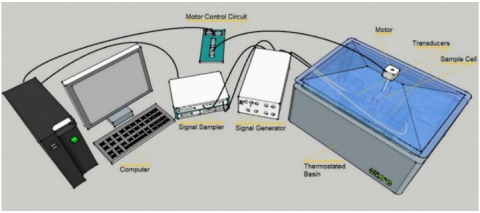Figure 1. Schematic presentation of ultrasound measurement system

The ultrasound system is composed of a hardware section and a software section. The hardware section is composed of several ultrasound instruments, as follow:

• Impulse generator (Signal Generator)
• Two immersion P-waves transducers of 0.5 MHz central frequency
• PC Oscilloscope (Signal Sampler)
• The sealed container (Sample Cell)
• Stepper motor with a resolution of 50 steps per degree
• Motor control circuit
• Room temperature (Thermostated basin)

## 2.png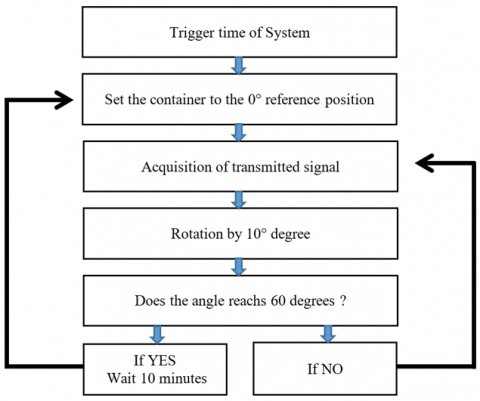Figure 2. The control operating graph of the container movement

The container is excited by plane wave pulses emitted at different angles, i.e., from 0° to 60°, by an immersion transducer. The whole experiment was finished after 50 h. As a recording interval, 10 min was taken. A Motor control circuit controls rotation of the sealed container. The stepper motor dynamics is controlled in a way that transmission pulse is generated after each 10° degree rotation, also matching the trigger time of the system. The movement of the container during the experiment is controlled following the operating graph below in Figure 2.

The aim of this present study is to measure longitudinal and transverse velocities of wave propagation into the cement mixture samples, which values are used to calculate Young’s modulus following the ultrasound measurement procedure below.

Generally, the measurement of longitudinal and transverse velocities requires to measure the time-of-Flight (ToF). Time-of-Flight (ToF) is one of the most important and pronounced features in a captured P-wave signal. The method of Time-of-Flight (ToF) principal is based on measurement of the time difference between two pulse echoes.

In our case, the measurement of both ultrasound velocities of wave propagation in cement paste needs first to measure the P-wave velocity in water and PMMA (Polymethacrylate) media. The wave propagation velocity in water is noted as

$V_w=\frac{D}{t_W}$                  (1)

where, $D$  is the distance respectively between the front surface of the transducer emitting the signal and the front surface of the transducer receiving the transmitted signal by the container, and $t_w$ , is the time-of-flight of the P-wave signal crossing the water between the two transducers, as shown in Figure 3.

## 3.png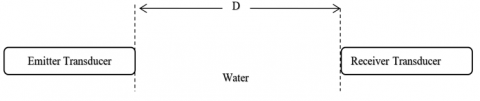Figure 3. The distance crossed by the P-wave between the emitter and the receiver transductions

Also, the wave propagation velocity in PMMA, denoted $V_{p l}$ , which depend on angle of rotation, is measured using the following formula,

$V_{p l}=\frac{\frac{e}{\cos \alpha}}{t_{p l}-\frac{D-\frac{e}{\cos \alpha}}{V_w}}$                  (2)

with $t_{p l}$ , the time-to-flight of the P-wave crossing water and PMMA media between the two transducers, as shown in Figure 4.

## 4.png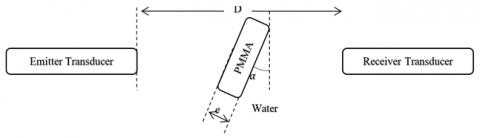Figure 4. The distance crossed by the P-wave in water and PMMA media between the two transducers

A general formulation of the ultrasound wave propagation velocity indicates its dependence on the rotation angle of the submerged container between the two immersion transducers. Thus, using this formula, the longitudinal velocity is calculated by choosing values of $\alpha$  between 0° and the limit angle which is less than 50°. For this range of values between 0° and 50°, both transverse and longitudinal waves are present. Therefore, to calculate the transverse velocity, an angle above the limit angle, which is less than 50°, should be chosen. Above 50°, the longitudinal mode is absent so that only the transverse mode is present.

The sealer parralipipeted container consists of two PMMA parallel face plates, delimiting the volume where the cement mixture is filled, as shown in Figure 5. The P-wave propagation velocity in the sample of cement mixture, isolated in the container, is therefore expressed as

$V_C=\frac{\frac{d}{\cos \alpha}}{t_C-\frac{D-\frac{2 e+d}{\cos \alpha}}{V_w}-\frac{\frac{2 e}{\cos \alpha}}{V_{p l}}}$                  (3)

## 5.png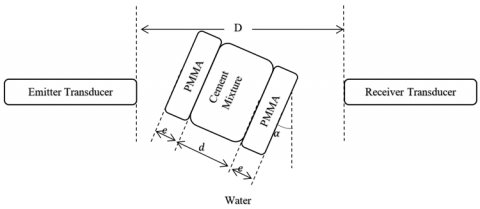Figure 5. The distance crossed by the P-wave in water and the sealed container between the two transducers

The velocity of propagation of ultrasound waves through the cement mixture sample in a given direction can be measured and related to the Young's modulus in that direction by the following equation

$E=\rho \times \frac{3 V_L^2-4 V_T^2}{V_L^2-V_T^2} \times V_T^2$                  (4)

where, $V_L$  Longitudinal wave velocity and $V_T$  transverse wave velocity as well as $\rho$  the cement density.

4. Results and Discussion

4.1 Effects of Temperature variation on cement paste

The variation of longitudinal and transverse wave velocities versus age of cement pastes, tested under curing different temperatures of 30°C, 35°C, 40°C and 45°C, are displayed respectively in Figures 6 and 7.

As presented in Figures 6 and 7, the gradual rise of curing temperature increases almost linearly both longitudinal and transversal velocities of ultrasound P-wave propagation in cement paste samples, during early ages of hydration. Both velocities curves show the same pattern. In all tested series 1 cement pastes, the ultrasound two velocities curves show a stepwise increase during the first 24 hours of hydration followed by the steady increase until reaching a stable value. The calculation of the two ultrasonic velocities started hours after the preparation of the cement paste since the Time-of-Flight (ToF) is difficult to measure during these first hours (pre-induction period, dormant period and part of the setting period), and in other terms, the captured echoes are difficult to process by the isolation algorithm during the first hours of hydration. The ultrasound longitudinal velocity evolves from values between 1000 m/s to 2000 m/s at a cement paste age between 4h and 7h to stable values during the early hardening period, between approximately 2600 m/s and 3100 m/s. The same evolution shape is remarked on the transversal velocity curves, where it evolves from values between 900 m/s to 1300 m/s at a cement paste age between 4h to 8h to stable values during the early hardening period, between 1550 m/s and 1650 m/s. However, stable values at early hardening period for longitudinal pulse velocity are more important than those of transversal pulse velocity. In all cases, it is clear that the ultrasound velocities calculations are sensitive to the effects of curing temperature variations on setting and early hardening hydration behaviour of cement pastes. A stepwise gradual increase of curing temperature by 5°C causes a gradual increase of both measured velocities, during the early ages of hydration. The hydraulic properties of cement-based materials during the hardening period, cured at elevated temperatures, was already studied in previous works [10, 11]. However, there are still few detailed investigations on the hydraulic behaviour during the early days of hydration and the development of the properties of cement pastes subjected to various curing temperatures. With increasing curing temperatures, the P-wave propagates faster in the cement paste medium during early hydration ages, which is shown in the results by higher values of both longitudinal and transversal velocities. The velocity of an ultrasound P-wave can be correlated and associated with changes in the microstructural properties of cement-based materials [12-14]. Thus, higher pulse velocities values are due to more and more interconnection between cement particles, and so, the cement material tested has a lower void volume [2, 15]. As mentioned in , and regarding the linearity relation between Young modulus and density, a cement material medium with lower void volume, i.e., a higher density [2, 16], has higher calculated Young modulus values. These results were validated since the stepwise increase of velocities with increasing curing temperature; provoke a stepwise increase of Young modulus values, as described in Figure 8.

## 6.pngFigure 6. Evolution of Longitudinal wave velocity vs cement pastes age, for series 1 cement mixes cured under different temperatures of 30°, 35°, 40° and 45°

## 7.png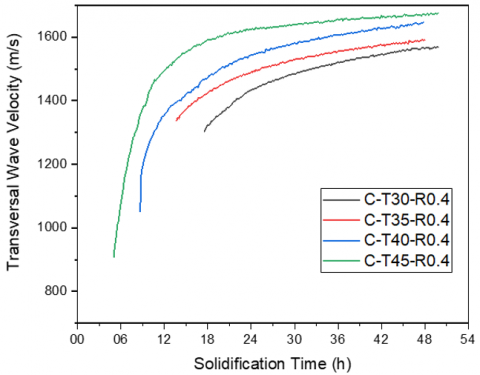Figure 7. Evolution of Transversal wave velocity vs cement pastes age, for series 1 cement mixes cured under different temperatures of 30°, 35°, 40° and 45°

## 8.png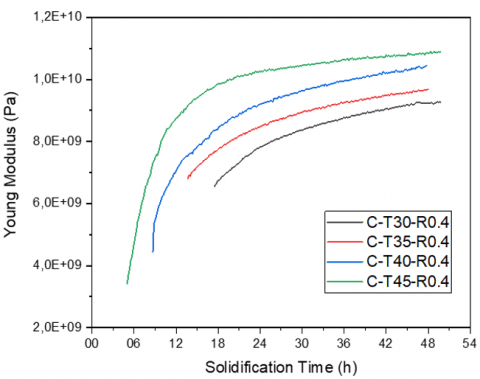Figure 8. Evolution of Young Modulus vs cement pastes age, for series 1 cement mixes cured under different temperatures of 30°, 35°, 40° and 45°

## 9.png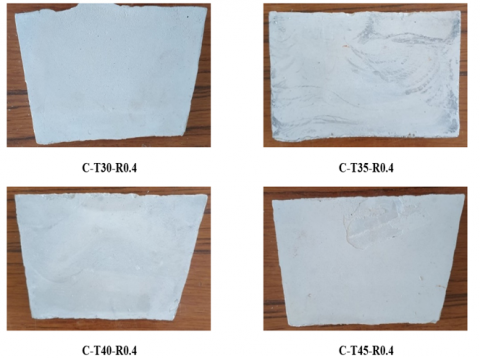Figure 9. Pictures of Series 1 Cement Samples with designation

Also, the time point at which both velocities begin to increase sharply provide important information about the hydration kinetics during the early age of cement paste. As shown in Figures 7 and 8, this time point occurs earlier as the curing temperature increases gradually. This means that a gradual increase of curing temperature causes a progressive loss of workability. With increasing curing temperature, the cement paste loses free water and part of absorbed water.

According to [16, 17], the rate of hydration of C3S is temperature dependent and increases with increasing temperature. Series 1 Cement Samples captured after 50h of hydration, are presented in Figure 9, with each one's designation.

4.2 Effects of water to cement ratios

The variation of longitudinal and transversal wave velocities versus age of cement pastes, prepared using water-to-cement ratios of 0.35, 0.40, 0.45 and 0.50 and cured under a curing temperature of 35 °C, are displayed respectively in Figures 10 and 11.

## 10.png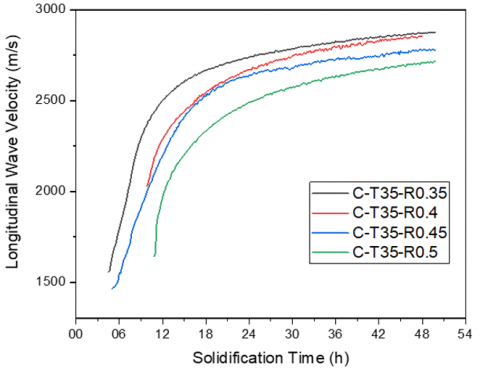Figure 10. Evolution of Longitudinal wave velocity vs cement pastes age, for series 2 cement mixes cured under a temperature of 35° with respect to water to cement ratios of 0.35, 0.4, 0.45 and 0.5

As shown in Figures 10 and 11, both ultrasound wave velocities are sensitive to the variation of water to cement (w/c) ratio. It can be seen from our results that lower w/c precipitates the accelerative increasing of wave velocities and earlier achievement of the steady state. This means that lower w/c leads to faster wave velocity development, and thus, faster setting and hardening of cement pastes . Clearly, a cement paste needs small amounts of water to bind solid phases together and form a more compact microstructure. When observing the different evolution curves of both wave velocities, we found that with decreasing w/c ratio, both longitudinal and transversal wave velocities values increase during the steady state of the early hardening period. This means that a cement paste with lower w/c ration presents a less attenuative medium. Zhang et al.  determined two characteristic time points to explain a correlation between pulse velocity evolution and early ages hydration behaviour of a cement material. The two characteristic points were used to classify the evolution of pulse velocity in cement material into three-hydration stage process. Using this method, Zhang et al.  prove that lower w/c ratio leads to a less attenuative cement paste medium and achieve higher pulse velocity values during the early ages of hydration. This may be explained partly by a decrease in void volume. In this context,  stated that the porosity of cement paste decreases with decreasing the w/c ratio. Zhang et al.  confirmed this statement by using 2D Sketches of the initial paste microstructure that show a lower initial solid volume fraction, i.e., a higher porosity, in a cement paste prepared with higher w/c ratio. In Trtnik et al. , a wave transmission method was proposed in order to monitor early ages hydration behaviour of an arbitrary cement paste mixture.

## 111.png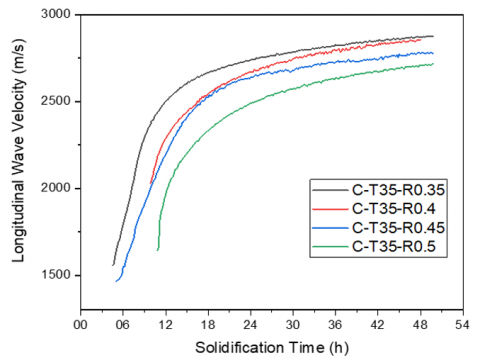Figure 11. Evolution of Longitudinal wave velocity vs cement pastes age, for series 2 cement mixes cured under a temperature of 35° with respect to water to cement ratios of 0.35, 0.4, 0.45 and 0.5

## 12.png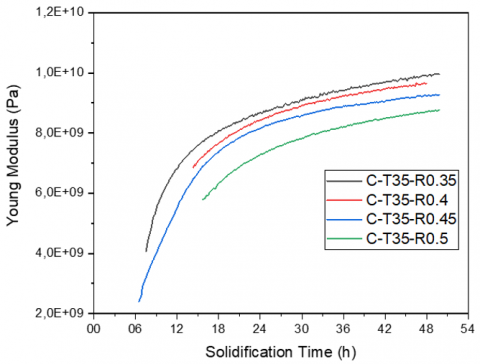Figure 12. Evolution of Young Modulus vs cement pastes age, for series 2 cement mixes cured under a temperature of 35° with respect to water to cement ratios of 0.35, 0.4, 0.45 and 0.5

## 13.png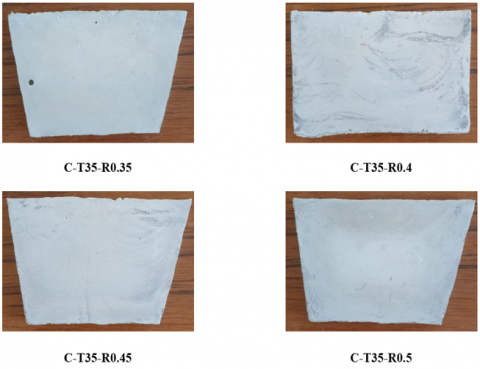Figure 13. Pictures of Series 2 Cement Samples with designation

This method used measurement of ultrasound longitudinal wave velocity to prove that lower w/c ratio results in shorter workability time. This phenomenon can be observed also using our newly developed method, from Figures 10 and 11.

Using Abram's generalization law [19, 20], Thanh  found that mechanical properties of concrete vary inversely with the w/c ratio. In Thanh's work , it was shown that with an increase in the water/cement ratio in concrete, the strength decreases. Thanh’s results were also validated for cement mortars [22-25]. In the same way, Young modulus is considered as a major mechanical property that describes the viscoelastic behaviour of cement pastes [6, 16]. Thus, our results showed that Young modulus decreases linearly with increasing w/c ratio, as represented in Figure 12. As mentioned by Zhang et al. , higher w/c ratio leads to higher porosity, which also can be, as presented by previous researches [26, 27], as a primary cause for drop of Young modulus values of cement pastes. Series 2 Cement Samples captured after 50h of hydration, are presented in Figure 13, with each one’s designation.

5. Conclusion

This study demonstrates a description of a creative newly developed non-destructive ultrasound method for early age hydration assessment of cement materials. This method is technically practical and is easily used so longitudinal and transverse velocities can be calculated and recorded for further analysis. This way the effects of different curing temperatures and various water to cement ratios on early hydration properties of cement pastes are characterized. Multiple resulted are induced for this experimental assessment:

1. The ultrasound longitudinal and transverse wave velocities are very sensitive to the effects of curing temperatures on early ages hydration of cement pastes
2. A stepwise increase of curing temperature causes a stepwise increase of both ultrasound wave velocities in cement pastes cured at 30°C, 35°C, 40°C and 45°C. Thus, Young modulus increases linearly with increasing curing temperature, during setting and early hardening periods of hydration.
3. The acoustic method used is highly sensitive in early ages of cement paste hydration and the difference of hydration behaviour caused by such small variations of w/c ratios can be clearly identified.
4. Lower w/c results in shorter workability time and leads to faster hardening of cement pastes.
5. Lower w/c ratio produces a less attenuative cement paste medium during the early hardening period, which can be partly due to a decrease in void volume.

Prepared cement pastes with a lower w/c ratio have a higher Young modulus during setting and early hardening periods of hydration. Thus, the Young modulus deceases linearly with the increase of w/c ratio.

References

 Lefever, G., Snoeck, D., De Belie, N., Van Vlierberghe, S., Van Hemelrijck, D., Aggelis, D.G. (2020). The contribution of elastic wave NDT to the characterization of modern cementitious media. Sensors, 20(10): 2959-2959. https://doi.org/10.3390/s20102959

 De Belie, N., Grosse, C.U., Kurz, J., Reinhardt, H.W. (2005). Ultrasound monitoring of the influence of different accelerating admixtures and cement types for shotcrete on setting and hardening behaviour. Cement and Concrete Research, 35(11): 2087-2094. https://doi.org/10.1016/j.cemconres.2005.03.011

 Reinhardt, H.W., Grosse, C.U. (2004). Continuous monitoring of setting and hardening of mortar and concrete. Construction and Building Materials, 18(3): 145-154. https://doi.org/10.1016/j.conbuildmat.2003.10.002

 Lotfi, H., bouazza, F.A.I.Z., Mesbah, H., Banouni, H. (2022). Spectral analysis of ultrasonic signals backscartted by mortar: Effect of sand size and temperatures. Materials Letters: X, 15: 100158-100158. https://doi.org/10.1016/j.mlblux.2022.100158

 Khatib, N., Hitar, M.E.H., Ouacha, E.H., Banouni, H., Faiz, B., Décultot, D., Ousbih, M. (2021). Monitoring early age behavior of mortar by ultrasound technique for environmental issues: Effects of sand grading and salinity of mixing water. Construction and Building Materials, 306: 124852-124852. https://doi.org/10.1016/j.conbuildmat.2021.124852

 Khatib, N., Ouacha, E., Faiz, B., Ezzaidi, M., Banouni, H. (2019). Analysis of the attenuative behaviour of accelerated cement based materials through a series of ultrasound Pulse Echo measurements. Engineering Solid Mechanics, 7(2): 109-120. https://doi.org/10.1016/10.5267/j.esm.2019.4.002

 Khatib, N., Ouacha, H., Faiz, B., Ezzaidi, M., Banouni, H. (2018). Ultrasound study of setting and hardening behaviour of mortar using Portland composite cements CPJ45 and CPJ35 and different dosages of an Alkalifree setting accelerator for Shotcrete. Journal of Engineering and Applied Sciences, 13: 7150-7155.

 Zhang, J., Fan, T., Ma, H., Li, Z. (2015). Monitoring setting and hardening of concrete by active acoustic method: effects of water-to-cement ratio and pozzolanic materials. Construction and Building Materials, 88: 118-125. https://doi.org/10.1016/j.conbuildmat.2015.04.010

 Banouni, H., Faiz, B., Ouacha, D.I.E.H., Boutaib, M., Derra, M. (2016). The canny edge detection method vs. the radius of curvature method for determining the time of flight on ultrasound. International Journal of Signal System Control and Engineering Application, 9(3): 48-54. https://doi.org/10.3923/ijssceapp.2016.48.54

 Alves, G.P., Jussiani, E.I., Andrello, A.C., Toralles, B.M. (2020). Effect of temperature rise on the microstructure of cementitious materials: A study through X-ray computed microtomography (µ-CT). Construction and Building Materials, 237: 117446-117446. https://doi.org/10.1016/j.conbuildmat.2019.117446

 Lion, M., Skoczylas, F., Lafhaj, Z., Sersar, M. (2005). Experimental study on a mortar. Temperature effects on porosity and permeability. Residual properties or direct measurements under temperature. Cement and Concrete Research, 35(10): 1937-1942. https://doi.org/10.1016/j.cemconres.2005.02.006

 Liao, C.M., Barroso, D.F. (2023). Investigation of temperature effects on ultrasonic velocity in a prestressed concrete bridge model. In European Workshop on Structural Health Monitoring, pp. 813-823. https://doi.org/10.1007/978-3-031-07258-1_82

 Jiang, J., Zhang, D., Gong, F., Zhi, D. (2022). Prediction of Ultrasonic Pulse Velocity for Cement, Mortar, and Concrete through a Multiscale Homogenization Approach. Materials, 15(9): 3241-3241. https://doi.org/10.3390/ma15093241

 Lofti, H., Faiz, B., Moudden, A., Menou, A., Izbaim, I., Maze, G., Decultot, D. (2009). Ultrasonic characterization and hardening of mortar using the reflection technique. High Temperature Materials and Processes, 28(4): 263-270. https://doi.org/10.1515/HTMP.2009.28.4.263

 Ye, G. (2003). Experimental study and numerical simulation of the development of the microstructure and permeability of cementitious materials. PhD thesis, T.U. Delft, The Netherlands.

 Hewlett, P., Liska, M. (2019). Lea's chemistry of cement and concrete. Butterworth-Heinemann.

 Klyusov, A.A. (1994). 3CaO SiO2 hydration under decreased temperatures. Cement and Concrete Research, 24(1): 127-132. https://doi.org/10.1016/0008-8846(94)90093-0

 Trtnik, G., Turk, G., Kavčič, F., Bosiljkov, V.B. (2008). Possibilities of using the ultrasonic wave transmission method to estimate initial setting time of cement paste. Cement and Concrete Research, 38(11): 1336-1342. https://doi.org/10.1016/j.cemconres.2008.08.003

 Abrams, D. A. (1919). Design of concrete mixtures. Structural Materials Research Laboratory, Lewis Institute, pp. 20-20.

 Popovics, S. (1981). Generalization of the abrams’ law-prediction of strength development of concrete from cement properties. In Journal Proceedings, 78(2): 123-129.

 Thanh, N.H. (1991). Optimal concrete composition based on paste content for ferrocement. Journal of Ferrocement, 21(4): 331-350.

 Rao, G.A. (2001). Generalization of Abrams' law for cement mortars. Cement and Concrete Research, 31(3): 495-502. https://doi.org/10.1016/S0008-8846(00)00473-7

 Singh, S.B., Munjal, P., Thammishetti, N. (2015). Role of water/cement ratio on strength development of cement mortar. Journal of Building Engineering, 4: 94-100. https://doi.org/10.1016/j.jobe.2015.09.003

 Haach, V.G., Vasconcelos, G., Lourenço, P.B. (2011). Influence of aggregates grading and water/cement ratio in workability and hardened properties of mortars. Construction and Building Materials, 25(6): 2980-2987. https://doi.org/10.1016/j.conbuildmat.2010.11.011

 Zhou, J., Chen, X., Wu, L., Kan, X. (2011). Influence of free water content on the compressive mechanical behaviour of cement mortar under high strain rate. Sadhana, 36(3): 357-369. https://doi.org/10.1007/s12046-011-0024-6

 Hasselman, D.P.H. (1963). Relation between effects of porosity on strength and on Young's modulus of elasticity of polycrystalline materials. Journal of the American Ceramic Society, 46(11): 564-565. https://doi.org/10.1111/j.1151-2916.1963.tb14615.x

 Yaman, I.O., Hearn, N., Aktan, H.M. (2002). Active and non-active porosity in concrete part I: experimental evidence. Materials and Structures, 35(2): 102-109. https://doi.org/10.1007/BF02482109